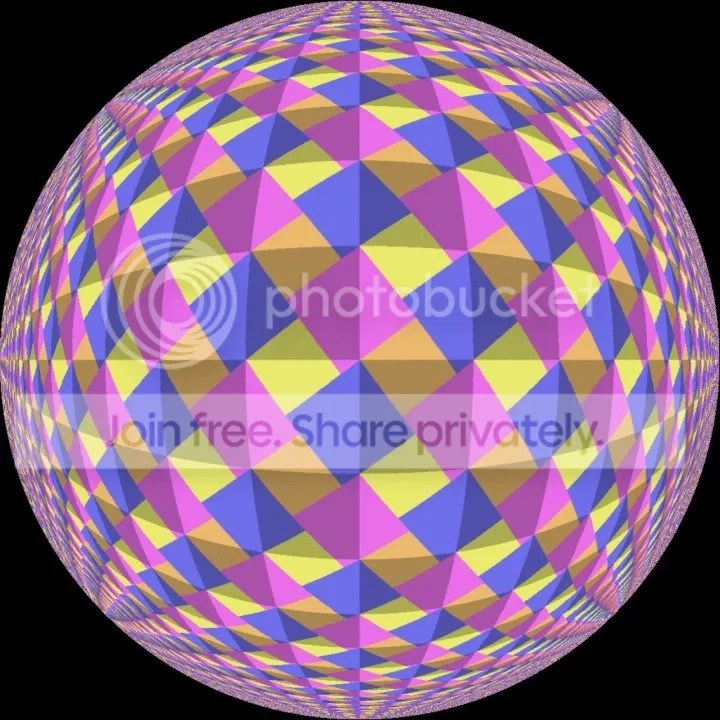## Animated Pythagoras

Two animatations of a “wordless” proof of Pythaoras’ Theorem provided by SSSF regular PM 2Ring.

This animation is a “wordless proof” of Pythagoras’ theorem.
The basic design is an interlocking pattern of coloured squares overlaid with “shadow” squares. In most of the animation frames, we have squares of 3 different sizes – there are big squares in two colours, small squares in two colours, and the shadow squares. By construction, all squares in a given group are identical in size. In some frames, the big squares and small squares are equal in size, and in some frames the small squares “degenerate” down to zero size, making the big coloured squares identical to the shadow squares. These special frames do not detract from the general argument.
In the general case, each side of a shadow square forms the hypotenuse of a right angle triangle, with a side of a small coloured square comprising the shortest side of the triangle, and a side of a big coloured square forming the remaining side.
The proof works by dissection. Inside any shadow square we have parts of three big coloured squares and parts of two small coloured squares. By symmetry and congruence the parts must match up. To be more explicit, there’s a triangular piece of one small square which is identical to the triangular piece that’s missing from the other small square. Thus these two pieces can be combined to make a whole small square. Similarly, the two triangular offcuts from big squares can be combined with the big square that has two triangular pieces missing. Hence the area of a shadow square is equal to the area of a small square plus the area of a big square. In other words the area of the square on the hypotenuse of the right angle triangle equals the sum of the areas of the squares on the other two sides, which is exactly what Pythagoras’ theorem states.
Here’s a link to a page that illustrates this proof.
http://fac-web.spsu.edu/math/tile/pythagorean/index.htm
This dissection was devised by Thabit ibn Qurra around 900 A.D.
I first saw this proof many years ago. I got the idea of animating it in the late 1980s or early 1990s, and wrote a program on the Amiga to do so.

PM 2RingAlso see this page for other (non-animated) versions of this tiling, including one projected onto the Riemann sphere.

Pythagoras, Penrose and Pov-Ray

And here’s a stereographic projection, reminiscent of Escher’s Circle Limit pictures.All images are the work of PM 2Ring.

This entry was posted in Animation, Maths, Newton and tagged , , , . Bookmark the permalink.

### 4 Responses to Animated Pythagoras

1.Georg says:

Hi Doug, nice stuff!! Everybody who likes interactive geometry might want to give GeoGebra a try (www.geogebra.org). In the current version 4, this Java-based software allows for saving sketches that are driven by a periodically varying pattern to be saved as animated GIFs. These can be easily recoded into FLCs suitable for upload to YouTube. It’s really fun!

Like

2.dougaj4 says:

I’ll have a look at that thanks Georg.

There should be a javascript version coming shortly as well!

Like

This site uses Akismet to reduce spam. Learn how your comment data is processed.